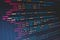# 10 Ways You Can Create Tensors In PyTorchPhoto by Florian Olivo on Unsplash

PyTorch is an open-source Python-based library. It provides high flexibility and speed while building, training, and deploying deep learning models.

At its core, PyTorch involves operations involving tensors. A tensor is a number, vector, matrix, or any n-dimensional array.

In this article, we will see different ways of creating tensors using PyTorch tensor methods (functions).

# tensor()

It returns a tensor when `data` is passed to it. `data` can be a scalar, tuple, a list, or a NumPy array.

In the above example, a NumPy array created using `np.arange()`

# Pointers In C — Definition, Notation, Types and Arithmetic

Pointers are arguably the most difficult feature of C to understand. But, it is one of the features which make C an excellent language. In a series of articles, we will take a closer look at pointers and how they can be used in C programming.

# Topics -

0. What exactly are pointers?
1. Pointer Notation and Definition
2. Some Special Pointers
3. Pointer Arithmetic

# 0. What exactly are pointers?

Before we go to the definition of pointers, let us understand what happens when we write

`int digit = 42;`

A block of memory is reserved by the compiler to hold an `int` value. The name of…## Srijan

Sachin, Cricket, Dhoni, ABD, Buttler